Fractions – 4 Worksheets

Fraction

Fraction Circles

Fraction Circles Template

Fraction Model

Fraction Subtraction

Fractions – Coloring

Fractions – Comparing

Fractions – Equivalent

Fractions – Halves

What is the fraction of the shaded part?Fraction

Fraction Circles

Fraction Circles Template

Fraction Model

Fraction Subtraction

Fractions – Coloring

Fractions – Comparing

Fractions – Equivalent

Fractions – Halves

### New Worksheets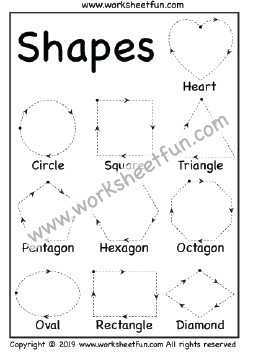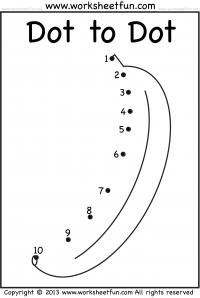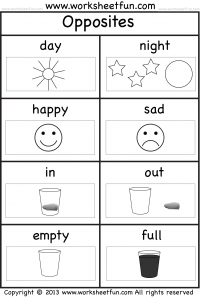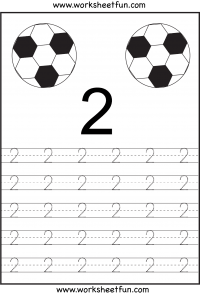### Most Popular Preschool and Kindergarten Worksheets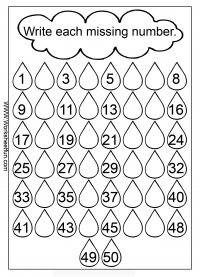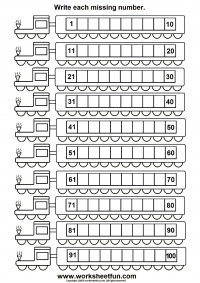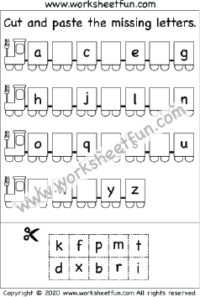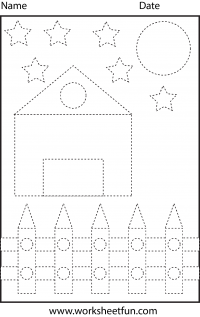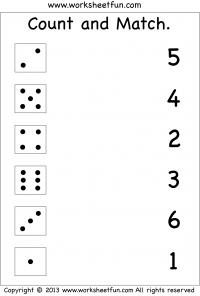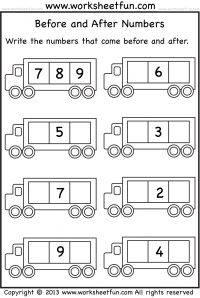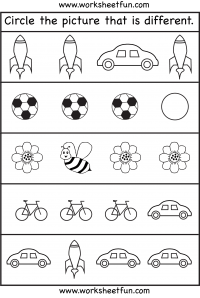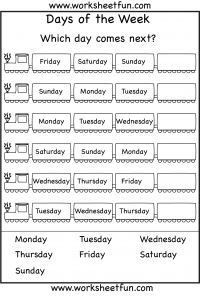#### Kindergarten Worksheets